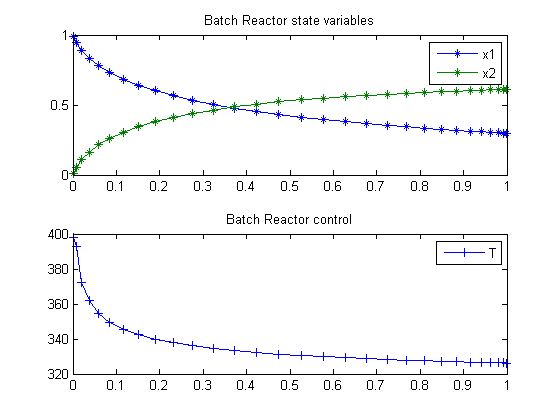13  Batch Reactor Problem

Example 6: DYNOPT User’s Guide version 4.1.0

Batch reactor with reactions: A -> B -> C.

M. Cizniar, M. Fikar, M. A. Latifi, MATLAB Dynamic Optimisation Code DYNOPT. User’s Guide, Technical Report, KIRP FCHPT STU Bratislava, Slovak Republic, 2006.

13.1  Problem description

Find T over t in [0; 1 ] to maximize

 J = x2(tf)

subject to:

 dx1 dt
= −k1*x12
 dx2 dt
= k1*x12k2*x2
k1 = 4000*exp
 2500 T

k2 = 620000*exp
 5000 T

where

 x(0) = [1  0]
 298 <= T <= 398

Reference: 

13.2  Problem setup

toms t
p = tomPhase('p', t, 0, 1, 30);
setPhase(p);

tomStates x1 x2
tomControls T

% Initial guess
% Note: The guess for t_f must appear in the list before expression involving t.
x0 = {icollocate({x1 == 1; x2 == 0})
collocate(T==398-t*100)};

% Box constraints
cbox = {298 <= collocate(T) <= 398};

% Boundary constraints
cbnd = initial({x1 == 1; x2 == 0});

% Various constants and expressions
k1 = 4000*exp(-2500./T);
k2 = 620000*exp(-5000./T);

% ODEs and path constraints
ceq = collocate({dot(x1) == -k1.*x1.^2
dot(x2) == k1.*x1.^2-k2.*x2});

% Objective
objective = -final(x2);

13.3  Solve the problem

options = struct;
options.name = 'Batch Reactor';
solution = ezsolve(objective, {cbox, cbnd, ceq}, x0, options);

% Extract optimal states and controls from solution
t  = subs(collocate(t),solution);
x1 = subs(collocate(x1),solution);
x2 = subs(collocate(x2),solution);
T  = subs(collocate(T),solution);
Problem type appears to be: lpcon
Starting numeric solver
===== * * * =================================================================== * * *
TOMLAB - Tomlab Optimization Inc. Development license  999001. Valid to 2011-02-05
=====================================================================================
Problem: ---  1: Batch Reactor                  f_k      -0.610799380695553730
sum(|constr|)      0.000006007956267540
f(x_k) + sum(|constr|)     -0.610793372739286240
f(x_0)      0.000000000000000000

Solver: snopt.  EXIT=0.  INFORM=1.
SNOPT 7.2-5 NLP code
Optimality conditions satisfied

FuncEv    1 ConstrEv   26 ConJacEv   26 Iter   23 MinorIter   84
CPU time: 0.093750 sec. Elapsed time: 0.094000 sec.

13.4  Plot result

subplot(2,1,1)
plot(t,x1,'*-',t,x2,'*-');
legend('x1','x2');
title('Batch Reactor state variables');

subplot(2,1,2)
plot(t,T,'+-');
legend('T');
title('Batch Reactor control');